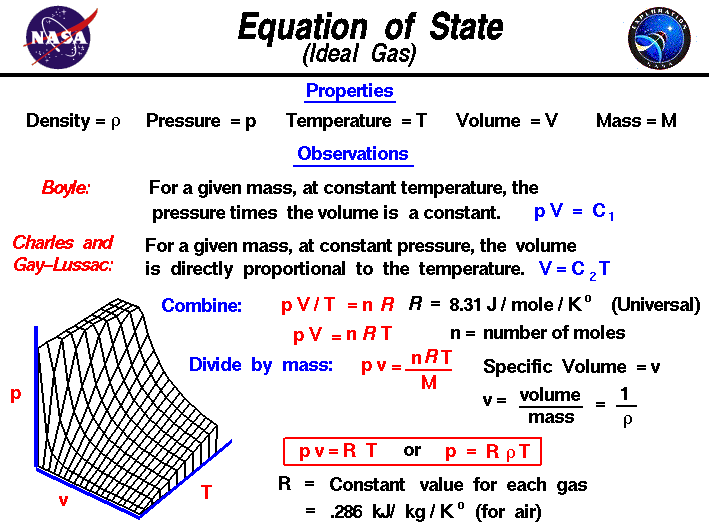+ Text Only Site
+ Non-Flash Version
+ Contact GlennGases have various properties that we can observe with our senses, including the gas pressure p, temperature T, mass m, and volume V that contains the gas. Careful, scientific observation has determined that these variables are related to one another, and the values of these properties determine the state of the gas. If we fix any two of the properties we can determine the nature of the relationship between the other two. You can explore the relationship between the variables at the animated gas lab. If the pressure and temperature are held constant, the volume of the gas depends directly on the mass, or amount of gas. This allows us to define a single additional property called the gas density r, which is the ratio of mass to volume. If the mass and temperature are held constant, the product of the pressure and volume are observed to be nearly constant for a real gas. The product of pressure and volume is exactly a constant for an ideal gas. This relationship between pressure and volume is called Boyle's Law in honor of Robert Boyle who first observed it in 1660. Finally, if the mass and pressure are held constant, the volume is directly proportional to the temperature for an ideal gas. This relationship is called Charles and Gay-Lussac's Law in honor of the two French scientists who discovered the relationship. The gas laws of Boyle and Charles and Gay-Lussac can be combined into a single equation of state given in red at the center of the slide: p * V / T = n * R where * denotes multiplication and / denotes division. To account for the effects of mass, we have defined the constant to contain two parts: a universal constant R and the mass of the gas expressed in moles n. Performing a little algebra, we obtain the more familiar form: p * V = n * R * T A three dimensional graph of this equation is shown at the lower left. The intersection point of any two lines on the graph gives a unique state for the gas. Engineers use a slightly different form of the equation of state that is specialized for a particluar gas. If we divide both sides of the general equation by the mass of the gas, the volume becomes the specific volume, which is the inverse of the gas density. We also define a new gas constant R, which is equal to the universal gas constant divided by the mass per mole of the gas. The value of the new constant depends on the type of gas as opposed to the universal gas constant, which is the same for all gases. The value of the equation of state for air is given on the slide as .286 kilo Joule per kilogram per degree Kelvin. The equation of state can be written in terms of the specific volume or in terms of the air density as p * v = R * T p = r * R * T Notice that the equation of state given here applies only to an ideal gas, or a real gas that behaves like an ideal gas. There are in fact many different forms for the equation of state for different gases. Also be aware that the temperature given in the equation of state must be an absolute temperature that begins at absolute zero. In the metric system of units, we must specify the temperature in degrees Kelvin (not Celsius). In the English system, absolute temperature is in degrees Rankine (not Fahrenheit). Guided ToursGas Statics:Speed of Sound:Animated Gas Lab:Activities: Equation of State (Ideal Gas) Activity: Grade 9-12 Related Sites: Rocket Index Rocket Home Beginner's Guide Home+ Inspector General Hotline + Equal Employment Opportunity Data Posted Pursuant to the No Fear Act + Budgets, Strategic Plans and Accountability Reports + Freedom of Information Act + The President's Management Agenda + NASA Privacy Statement, Disclaimer, and Accessibility CertificationEditor: Tom Benson NASA Official: Tom Benson Last Updated: May 13 2021 + Contact Glenn